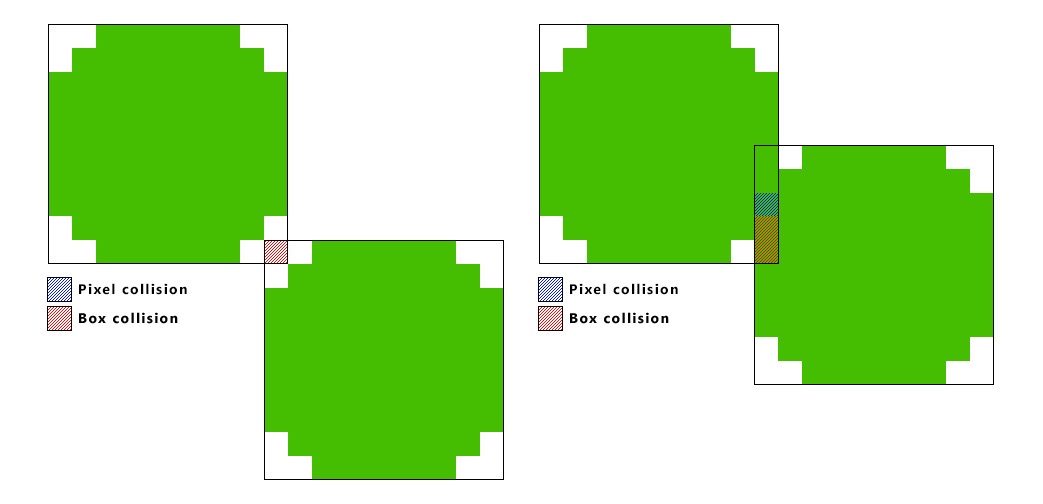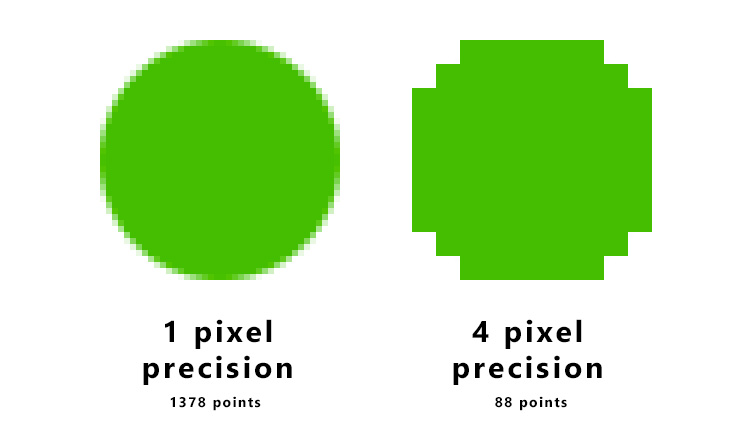# 使用JavaScript和canvas做精确的像素碰撞检测

    /* 描述像素图的伪代码 */
var pixelMap = [];
for( var y = 0; y < image.width; y++ ) {
for( var x = 0; x < image.height; x++ ) {
// 获取当前位置的元素
var pixel = ctx.getImageData( x, y, 1, 1 );
// 判断透明度不为0
if( pixel.data != 0 ) {
pixelMap.push( { x:x, y:y } );
}
}
}
return pixelMap;

    /* 盒模型测试， 碰撞返回 true */
function hitBox( source, target ) {
/* 源物体和目标物体都包含 x, y 以及 width, height */
return !(
( ( source.y + source.height ) < ( target.y ) ) ||
( source.y > ( target.y + target.height ) ) ||
( ( source.x + source.width ) < target.x ) ||
( source.x > ( target.x + target.width ) )
);
}/* 像素碰撞检测的伪代码 */
function pixelHitTest( source, target ) {
// 循环源图像的所有像素
for( var s = 0; s < source.pixelMap.length; s++ ) {
var sourcePixel = source.pixelMap[s];
// 添加位置偏移
var sourceArea = {
x: sourcePixel.x + source.x,
y: sourcePixel.y + source.y,
width: 1,
height: 1
};

// 循环目标图像的所有像素
for( var t = 0; t < target.pixelMap.length; t++ ) {
var targetPixel = target.pixelMap[t];
// 添加位置偏移
var targetArea = {
x: targetPixel.x + target.x,
y: targetPixel.y + target.y,
width: 1,
height: 1
};

/* 使用之前提到的 hitbox 函数 */
if( hitBox( sourceArea, targetArea ) ) {
return true;
}
}
}
}/* 描绘更大分辨率像素图的伪代码 */
function generateRenderMap( image, resolution ) {
var pixelMap = [];
for( var y = 0; y < image.width; y=y+resolution ) {
for( var x = 0; x < image.height; x=x+resolution ) {
// 获取当前位置的像素群
var pixel = ctx.getImageData( x, y, resolution, resolution );

// 判断像素群的透明度不为0
if( pixel.data != 0 ) {
pixelMap.push( { x:x, y:y } );
}
}
}
return {
data: pixelMap,
resolution: resolution
};
}

/* 像素碰撞测试伪代码 */
function pixelHitTest( source, target ) {

// 源对象和目标对象包含两张属性
// { data: a render-map, resolution: The precision of the render-map}

// 循环源对象的所有像素
for( var s = 0; s < source.pixelMap.data.length; s++ ) {
var sourcePixel = source.data.pixelMap[s];
// 添加位置偏移
var sourceArea = {
x: sourcePixel.x + source.x,
y: sourcePixel.y + source.y,
width: target.pixelMap.resolution,
height: target.pixelMap.resolution
};

// 循环源对象的所有像素
for( var t = 0; t < target.pixelMap.data.length; t++ ) {
var targetPixel = target.pixelMap.data[t];
// 添加位置偏移
var targetArea = {
x: targetPixel.x + target.x,
y: targetPixel.y + target.y,
width: target.pixelMap.resolution,
height: target.pixelMap.resolution
};

/*使用之前提到的 hitbox 函数 */
if( hitBox( sourceArea, targetArea ) ) {
return true;
}
}
}
}